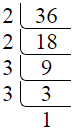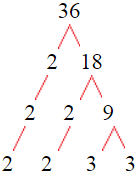# Factors of 36

The factors of 36 are 1, 2, 3, 4, 6, 9, 12, 18, and 36 i.e. F36 = {1, 2, 3, 4, 6, 9, 12, 18, 36}. The factors of 36 are all the numbers that can divide 36 without leaving a remainder.

We can check if these numbers are factors of 36 by dividing 36 by each of them. If the result is a whole number, then the number is a factor of 36. Let's do this for each of the numbers listed above:

·        1 is a factor of 36 because 36 divided by 1 is 36.

·        2 is a factor of 36 because 36 divided by 2 is 18.

·        3 is a factor of 36 because 36 divided by 3 is 12.

·        4 is a factor of 36 because 36 divided by 4 is 9.

·        6 is a factor of 36 because 36 divided by 6 is 6.

·        9 is a factor of 36 because 36 divided by 9 is 4.

·        12 is a factor of 36 because 36 divided by 12 is 3.

·        18 is a factor of 36 because 36 divided by 18 is 2.

·        36 is a factor of 36 because 36 divided by 36 is 1.

## How to Find Factors of 36?

1 and the number itself are the factors of every number. So, 1 and 36 are two factors of 36. To find the other factors of 36, we can start by dividing 36 by the numbers between 1 and 36. If we divide 36 by 2, we get a remainder of 0. Therefore, 2 is a factor of 36. If we divide 36 by 3, we get a remainder of 0. Therefore, 3 is also a factor of 36.

Next, we can check if 4 is a factor of 36. If we divide 36 by 4, we get a remainder of 0. Therefore, 4 is also a factor of 36. If we divide 36 by 5, we get a remainder of 1. Therefore, 5 is not a factor of 36. We can continue this process for all the possible factors of 36.

Through this process, we can find that the factors of 36 are 1, 2, 3, 4, 6, 9, 12, 18, and 36. These are the only numbers that can divide 36 without leaving a remainder.

********************

********************

## Properties of the Factors of 36

The factors of 36 have some interesting properties. One of the properties is that the sum of the factors of 36 is equal to 91. We can see this by adding all the factors of 36 together:

1 + 2 + 3 + 4 + 6 + 9 + 12 + 18 + 36 = 91

Another property of the factors of 36 is that the prime factors of 36 are 2 and 3 only.

## Applications of the Factors of 36

The factors of 36 have several applications in mathematics. One of the applications is in finding the highest common factor (HCF) of two or more numbers. The HCF is the largest factor that two or more numbers have in common. For example, to find the HCF of 36 and 42, we need to find the factors of both numbers and identify the largest factor they have in common. The factors of 36 are 1, 2, 3, 4, 6, 9, 12, 18, and 36. The factors of 42 are 1, 2, 3, 6, 7, 14, 21, and 42. The largest factor that they have in common is 6. Therefore, the HCF of 36 and 42 is 6.

Another application of the factors of 36 is in prime factorization. Prime factorization is the process of expressing a number as the product of its prime factors. The only prime factors of 36 are 2 and 3. We can express 36 as:

36 = 2 × 2 × 3 × 3

We can do prime factorization by division and factor tree method also. Here is the prime factorization of 36 by division method,36 = 2 × 2 × 3 × 3

Here is the prime factorization of 36 by the factor tree method,36 = 2 × 2 × 3 × 3

## Conclusion

The factors of 36 are the numbers that can divide 36 without leaving a remainder. The factors of 36 are 1, 2, 3, 4, 6, 9, 12, 18, and 36. The factors of 36 have some interesting properties, such as having a sum of 91. The factors of 36 have several applications in mathematics, such as finding the highest common factor and prime factorization.